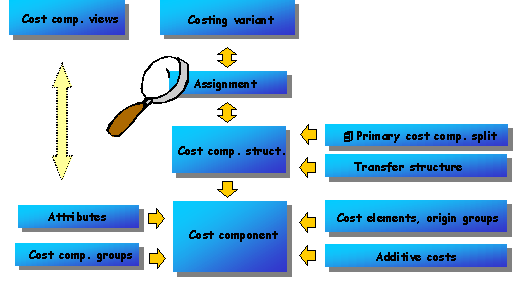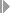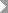Show TOC

###Cost Component#### Definition

Grouping of cost elements with or without origin groups.

#### Use

The costs from a cost estimate are assigned to cost elements and cost components. You can use the origin groups in the material master records to subdivide the material costs within a cost element.

You can use cost components to specify that costs should be included in the relevant inventory valuation, for example.

You create origin groups and cost components for in Customizing for Product Cost Planning under Basic Settings for Material Costing . Based on the cost components that you have defined in Customizing, you can do the following:

• Create cost component views that contain costs such as the cost of goods manufactured, sales and administration costs or the costs for inventory valuation

• Group cost components differently according to the purpose for which costing was carried out (such as stock valuation or inventory valuation)

#### Integration

The definition of the cost components in Customizing for Product Cost Planning determines how the costed material is valuated. For each cost component, you specify whether the assigned costs are included with the following valuations :

• Inventory valuation

• Physical inventory valuation based on commercial law

• Physical inventory valuation based on tax law

• Transfer price surcharge

For each valuation, you define the relevant proportion of the costs:

• You flag the cost component as not relevant .

This prevents certain costs (such as production overhead) from being used in inventory costing.

• You flag the cost component as variable costs .

This means that only the variable portion of certain costs (such as internal activities) are used in inventory costing.

• You flag the cost component as fixed and variable costs .

This means that the full costs (such as for raw materials) are used in stock valuation.

You also specify the following for each cost component:

• Whether the costs assigned to the cost component are to be treated as the cost of goods manufactured

• Whether the costs assigned to the cost component are included in an initial cost split (a cost component split for raw materials). You can create an additive cost estimate , to include freight charges and insurance costs for raw materials. Alternatively, you can create a raw material cost estimate .

• Whether delta profits (profits between company codes and profit centers) should be updated. This indicator must be set when you create a group cost estimate .

These settings are then included when the costing data is transferred into the material master record.

Type of cost estimate whose results are transferred to the material master

Type of valuation

Resulting price

Standard Cost Estimates

Inventory valuation

Future planned price

Inventory cost estimate

Physical inventory based on commercial law

Price based on commercial law

Inventory based on tax law

Price based on tax law

If you transfer the results of a modified standard cost estimate or a current cost estimate into the material master record, you can specify which cost component view should be transferred.

#### Features

The following graphic illustrates how cost components, cost component structures, and cost views are customized:Cost components are grouped into a cost component structure. A cost component structure can have up to 40 cost components. However, if the cost components contain both fixed and variable costs, the number of costs components is limited to 20.

Examples of cost components are:

• Raw materials

• Personnel costs

• Production costs

• External activities

• Other costs

If you are using a particular costing variant, the system determines the cost component structure for this costing variant and creates the cost component split for the costing results accordingly.Note

All costing variants for the standard cost estimate in a company code must be linked to the same cost component structure. Otherwise you cannot transfer costing results from other plants for specially-procured materials.

For costing variants that are not set for the standard cost estimate, you can assign the cost component structure separately for each plant or for each costing variant.

End of the note.Note

The values for each cost component are updated in the currency of the company code to which the material is assigned.

End of the note.

For further information about defining cost components, see the Implementation Guide (IMG) for Product Cost Controlling underProduct Cost PlanningBasic Settings for Material CostingDefine Cost Components.